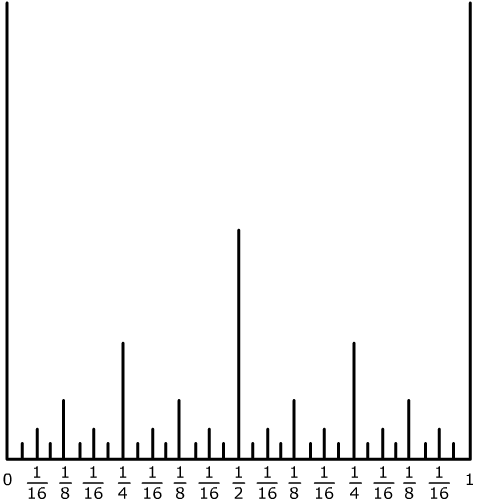#### You may also like### Converging Product

In the limit you get the sum of an infinite geometric series. What about an infinite product (1+x)(1+x^2)(1+x^4)... ?A circle is inscribed in an equilateral triangle. Smaller circles touch it and the sides of the triangle, the process continuing indefinitely. What is the sum of the areas of all the circles?### Binary Squares

If a number N is expressed in binary by using only 'ones,' what can you say about its square (in binary)?

# Ruler

##### Age 16 to 18Challenge Level

Andaleeb sent in this excellent solution.

The diagram shows some of the vertical lines drawn for values of x between 0 and 1 as described in the question. The lines are of height 1 unit at x = 0 and 1, of height ${1\over 2}$ units at $x= {1\over 2}$ , of height ${1\over 4}$ units at $x= {k\over 4}$ and ${1\over 8}$ units at $x= {k\over 8}$ and so on... up to ${1\over 2^5}$ at ${k\over 2^5}$ where $k$ is a positive integer.

 n $0$ $1$ $2$ $3$ $4$ $5$ $6$ $\dots$ $n$ $n+1$ Height $1$ ${1\over 2}$ ${1\over 4 }$ ${1\over 8}$ ${1\over 16}$ ${1\over 32 }$ ${1\over 64}$ $\dots$ ${1\over 2^n}$ ${1\over 2^{n+1`}}$ Lines cut $2$ $1$ $2$ $4$ $8$ $16$ $32$ $\dots$ $2^{n-1}$ $2^n$Thus if the height $h$ lies in ${1\over 2^n} > h> {1\over 2^{n+1}}$ then the number of lines cut is given by $$2 + 1 + 2 + 4 + 8 + ... + 2^{n-1} = 2 + {{2^n - 1} \over {2 - 1}} = 2^n + 1.$$ As $n$ tends to infinity the height of the lines tends to 0 and the number of lines cut tends to infinity.